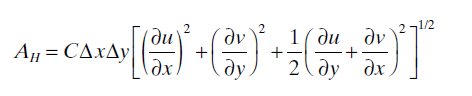# Turbulent diffusion option

Hello,

I have questions about the calcuation method of horizontal eddy viscosity in EFDC.

As I know, there are three options to calculate horizontal eddy viscosity (=AH).
From other postings, I understand that the first option “Disable HMD” uses a constant AH (AH = AHO) and the second option “Activate HMD with Smagorinsky” uses time varying AH (AH = AHO + result of smagorinsky model with AHD).

But, I don’t undertstand the third option (Activate HMD with Smagorinsky, Wall Drag and WC Diffusion).
I’d like to know what is the role of “wall drag and WC diffusion” to calculate the AH.
And, what is the difference between the second option and the third option?

Thank you.

Hi,
Smagorinsky coefficient is calculated using the following formulation in EFDC model:The difference between second and third option is essentially the computation of wall drag and WC diffusion. In the third option, the computation of du/dy and dv/dx is different than the second option. The second option doesn’t use the effects of wall drag and WC diffusion.
Bottom drag coefficient is calculated as:where k = 0.4 = von Karman’s constant,
delzb = dimensionless thickness of bottom layer ,
zo = dimensionless roughness heights ,
zo* = bottom roughness height

In the third option slip factor is computed as follows:
SLIPFAC = SLIPCO*SQRT(CSDRAG)
where CSDRAG = CB as shown above, SLIPCO = 0.5/SQRT(AHD)

Please check calhdmf.f90 for the calculations using the second and third option in detail.

Best,
Janesh Devkota

Thank you for your kind explanation.

According to your explanation, I tried to find a related document about the use of SLIPFAC to calculate velocity gradient.
But, I cannot find one.
Would you mind recommending me any document which explains about the use of SLIPFAC?

Also, I’d like to know more about the WC diffusion in the option 3 (Activate HMD with Smagorinsky, Wall Drag and WC Diffusion).
When I selected the option 3, I can find that the concentration slope was decreased as increasing AHO or AHD.
It looks like that the horizontal diffusion coefficient used in the mass transport equation (DYE module) was increased with increasing AHO or AHD.
But, I cannot get this results when I selected the option 2 (Activate HMD with Smagorinsky).
Do I have to select the option 3 if I want to manipulate the concentration slope of DYE module simulation results?
If I need to select the option 3, how does the option 3 affect to the horizontal diffusion coefficient in the mass transport equation?
Does it assume that the horizontal eddy viscosity is same as the horizontal diffusion coefficient according to the Reynolds analogy only when I select the option 3?

Thank you.